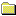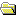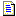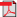Products Download Events Support Videos All Product Families ARM7, ARM9, and Cortex-M3 Products C16x, XC16x, and ST10 Products C251 and 80C251 Products Cx51 and 8051 Products Modified Anytime In the Last 7 Days In the Last 14 Days In the Last 30 Days In the Last 90 Days In the Last 6 Months

Technical Support

On-Line Manuals

Compiler Reference GuidePrefaceArm Compiler Tools Overviewarmclang Referencearmlink Referencefromelf Referencearmar Referencearmasm Legacy Assembler ReferenceAppendixesStandard C Implementation DefinitionImplementation definitionTranslationTranslation limitsEnvironmentIdentifiersCharactersIntegersFloating-pointArrays and pointersHintsStructures, unions, enumerations, and bitfieldsQualifiersPreprocessing directivesLibrary functionsArchitectureStandard C++ Implementation DefinitionImplementation definitionGeneralLexical conventionsBasic conceptsStandard conversionsExpressionsDeclarationsDeclaratorsTemplatesException handlingPreprocessing directivesLibrary introductionLanguage support libraryGeneral utilities libraryStrings libraryNumerics libraryLocalization libraryContainers libraryInput/output libraryRegular expressions libraryAtomic operations libraryThread support libraryImplementation quantitiesVia File SyntaxOverview of via filesVia file syntax rules

# <!-- var kmNextPage = "armclang_ref_lft1506441785512.htm"; var kmPrevPage = "armclang_ref_yug1493281506044.htm"; var kmNextPageTitle = "Standard C++ Implementation Definition"; var kmPrevPageTitle = "Library functions"; var kmBreadCrumbs = "<a href=\"default.htm\">Home</a> &raquo; <a href=\"armclang_ref_fyk1564498919356.htm\">Appendixes</a> &raquo; <a href=\"armclang_ref_pvk1493280214273.htm\">Standard C Implementation Definition</a> &raquo; Architecture"; kmSetupPaging (); kmNavButtons(); //--> Architecture

## A.15 Architecture

Describes implementation-defined aspects of the Arm® C compiler and C library relating to architecture, as required by the ISO C standard.

The values or expressions assigned to the macros specified in the headers `<float.h>`, `<limits.h>`, and `<stdint.h>` (5.2.4.2, 7.20.2, 7.20.3).

### Note:

If the value column is empty, this means no value is assigned to the corresponding macro.

The values of the macros in `<float.h>` are:

Macro name Value
`FLT_ROUNDS` `1`
`FLT_EVAL_METHOD` `0`
`FLT_HAS_SUBNORM`
`DBL_HAS_SUBNORM`
`LDBL_HAS_SUBNORM`
`FLT_RADIX` `2`
`FLT_MANT_DIG` `24`
`DBL_MANT_DIG` `53`
`LDBL_MANT_DIG` `53`
`FLT_DECIMAL_DIG`
`DBL_DECIMAL_DIG`
`LDBL_DECIMAL_DIG`
`DECIMAL_DIG` `17`
`FLT_DIG` `6`
`DBL_DIG` `15`
`LDBL_DIG` `15`
`FLT_MIN_EXP` `(-125)`
`DBL_MIN_EXP` `(-1021)`
`LDBL_MIN_EXP` `(-1021)`
`FLT_MIN_10_EXP` `(-37)`
`DBL_MIN_10_EXP` `(-307)`
`LDBL_MIN_10_EXP` `(-307)`
`FLT_MAX_EXP` `128`
`DBL_MAX_EXP` `1024`
`LDBL_MAX_EXP` `1024`
`FLT_MAX_10_EXP` `38`
`DBL_MAX_10_EXP` `308`
`LDBL_MAX_10_EXP` `308`
`FLT_MAX` `3.40282347e+38F`
`DBL_MAX` `1.79769313486231571e+308`
`LDBL_MAX` `1.79769313486231571e+308L`
`FLT_EPSILON` `1.19209290e-7F`
`DBL_EPSILON` `2.2204460492503131e-16`
`LDBL_EPSILON` `2.2204460492503131e-16L`
`FLT_MIN` `1.175494351e-38F`
`DBL_MIN` `2.22507385850720138e-308`
`LDBL_MIN` `2.22507385850720138e-308L`
`FLT_TRUE_MIN`
`DBL_TRUE_MIN`
`LDBL_TRUE_MIN`

The values of the macros in `<limits.h>` are:

Macro name Value
`CHAR_BIT` `8`
`SCHAR_MIN` `(-128)`
`SCHAR_MAX` `127`
`UCHAR_MAX` `255`
`CHAR_MIN` `0`
`CHAR_MAX` `255`
`MB_LEN_MAX` `6`
`SHRT_MIN` `(-0x8000)`
`SHRT_MAX` `0x7fff`
`USHRT_MAX` `65535`
`INT_MIN` `(~0x7fffffff)`
`INT_MAX` `0x7fffffff`
`UINT_MAX` `0xffffffffU`
`LONG_MIN` `(~0x7fffffffL)`
`LONG_MIN (64-bit)` `(~0x7fffffffffffffffL)`
`LONG_MAX` `0x7fffffffL`
`LONG_MAX (64-bit)` `0x7fffffffffffffffL`
`ULONG_MAX` `0xffffffffUL`
`ULONG_MAX (64-bit)` `0xffffffffffffffffUL`
`LLONG_MIN` `(~0x7fffffffffffffffLL)`
`LLONG_MAX` `0x7fffffffffffffffLL`
`ULLONG_MAX` `0xffffffffffffffffULL`

The values of the macros in `<stdint.h>` are:

Macro name Value
`INT8_MIN` `-128`
`INT8_MAX` `127`
`UINT8_MAX` `255`
`INT16_MIN` `-32768`
`INT16_MAX` `32767`
`UINT16_MAX` `65535`
`INT32_MIN` `(~0x7fffffff)`
`INT32_MAX` `2147483647`
`UINT32_MAX ` `4294967295u`
`INT64_MIN` `(~0x7fffffffffffffffLL)`
`INT32_MAX` `2147483647`
`UINT32_MAX ` `4294967295u`
`INT64_MIN (64-bit)` `(~0x7fffffffffffffffL)`
`INT64_MAX (64-bit)` `(9223372036854775807L)`
`UINT64_MAX (64-bit)` `(18446744073709551615uL)`
`INT_LEAST8_MIN` `-128`
`INT_LEAST8_MAX` `127`
`UINT_LEAST8_MAX` `255`
`INT_LEAST16_MIN` `-32768`
`INT_LEAST16_MAX` `32767`
`UINT_LEAST16_MAX` `65535`
`INT_LEAST32_MIN` `(~0x7fffffff)`
`INT_LEAST32_MAX` `2147483647`
`UINT_LEAST32_MAX ` `4294967295u`
`INT_LEAST64_MIN` `(~0x7fffffffffffffffLL)`
`INT_LEAST64_MAX` `(9223372036854775807LL)`
`UINT_LEAST64_MAX` `(18446744073709551615uLL)`
`INT_LEAST64_MIN (64-bit)` `(~0x7fffffffffffffffL)`
`INT_LEAST64_MAX (64-bit)` `(9223372036854775807L)`
`UINT_LEAST64_MAX (64-bit)` `(18446744073709551615uL)`
`INT_FAST8_MIN` `(~0x7fffffff)`
`INT_FAST8_MAX` `2147483647`
`UINT_FAST8_MAX ` `4294967295u`
`INT_FAST16_MIN` `(~0x7fffffff)`
`INT_FAST16_MAX` `2147483647`
`UINT_FAST16_MAX ` `4294967295u`
`INT_FAST32_MIN` `(~0x7fffffff)`
`INT_FAST32_MAX` `2147483647`
`UINT_FAST32_MAX ` `4294967295u`
`INT_FAST64_MIN` `(~0x7fffffffffffffffLL)`
`INT_FAST64_MAX` `(9223372036854775807LL)`
`UINT_FAST64_MAX` `(18446744073709551615uLL)`
`INT_FAST64_MIN (64-bit)` `(~0x7fffffffffffffffL)`
`INT_FAST64_MAX (64-bit)` `(9223372036854775807L)`
`UINT_FAST64_MAX (64-bit)` `(18446744073709551615uL)`
`INTPTR_MIN` `(~0x7fffffff)`
`INTPTR_MIN (64-bit)` `(~0x7fffffffffffffffLL)`
`INTPTR_MAX` `2147483647`
`INTPTR_MAX (64-bit)` `(9223372036854775807LL)`
`UINTPTR_MAX` `4294967295u`
`UINTPTR_MAX (64-bit)` `(18446744073709551615uLL)`
`INTMAX_MIN` `(~0x7fffffffffffffffll)`
`INTMAX_MAX` `(9223372036854775807ll)`
`UINTMAX_MAX` `(18446744073709551615ull)`
`PTRDIFF_MIN` `(~0x7fffffff)`
`PTRDIFF_MIN (64-bit)` `(~0x7fffffffffffffffLL)`
`PTRDIFF_MAX` `2147483647`
`PTRDIFF_MAX (64-bit)` `(9223372036854775807LL)`
`SIG_ATOMIC_MIN` `(~0x7fffffff)`
`SIG_ATOMIC_MAX` `2147483647`
`SIZE_MAX` `4294967295u`
`SIZE_MAX (64-bit)` `(18446744073709551615uLL)`
`WCHAR_MIN` `0`
`WCHAR_MAX` `0xffffffffU`
`WINT_MIN` `(~0x7fffffff)`
`WINT_MAX` `2147483647`
The result of attempting to indirectly access an object with automatic or thread storage duration from a thread other than the one with which it is associated (6.2.4).
Access to automatic or thread storage duration objects from a thread other than the one with which the object is associated proceeds normally.
The number, order, and encoding of bytes in any object (when not explicitly specified in this International Standard) (6.2.6.1).
Defined in the Arm EABI.
Whether any extended alignments are supported and the contexts in which they are supported, and valid alignment values other than those returned by an `_Alignof` expression for fundamental types, if any (6.2.8).
Alignments, including extended alignments, that are a power of 2 and less than or equal to `0x10000000`, are supported.
The value of the result of the `sizeof` and `_Alignof` operators (6.5.3.4).
Type `sizeof` `_Alignof`
`char` `1` `1`
`short` `2` `2`
`int` `4` `4`
`long` (AArch32 state) `4` `4`
`long` (AArch64 state) `8` `8`
`long long` `8` `8`
`float` `4` `4`
`double` `8` `8`
`long double` (AArch32 state) `8` `8`
`long double` (AArch64 state) `16` `16`
 Non-ConfidentialPDF version 101754_0614_00_en Copyright © 2019, 2020 Arm Limited or its affiliates. All rights reserved.##### Important information

This site uses cookies to store information on your computer. By continuing to use our site, you consent to our cookies.